xFinite setEncyclopedia
In mathematics
Mathematics
Mathematics is the study of quantity, space, structure, and change. Mathematicians seek out patterns and formulate new conjectures. Mathematicians resolve the truth or falsity of conjectures by mathematical proofs, which are arguments sufficient to convince other mathematicians of their validity...

, a finite set is a set that has a finite number of elements. For example,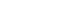is a finite set with five elements. The number of elements of a finite set is a natural number
Natural number
In mathematics, the natural numbers are the ordinary whole numbers used for counting and ordering . These purposes are related to the linguistic notions of cardinal and ordinal numbers, respectively...

(non-negative integer
Integer
The integers are formed by the natural numbers together with the negatives of the non-zero natural numbers .They are known as Positive and Negative Integers respectively...

), and is called the cardinality of the set. A set that is not finite is called infinite. For example, the set of all positive integers is infinite: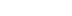Finite sets are particularly important in combinatorics
Combinatorics
Combinatorics is a branch of mathematics concerning the study of finite or countable discrete structures. Aspects of combinatorics include counting the structures of a given kind and size , deciding when certain criteria can be met, and constructing and analyzing objects meeting the criteria ,...

, the mathematical study of counting
Counting
Counting is the action of finding the number of elements of a finite set of objects. The traditional way of counting consists of continually increasing a counter by a unit for every element of the set, in some order, while marking those elements to avoid visiting the same element more than once,...

. Many arguments involving finite sets rely on the pigeonhole principle, which states that there cannot exist an injective function
Injective function
In mathematics, an injective function is a function that preserves distinctness: it never maps distinct elements of its domain to the same element of its codomain. In other words, every element of the function's codomain is mapped to by at most one element of its domain...

from a larger finite set to a smaller finite set.

## Definition and terminology

Formally, a set S is called finite if there exists a bijection
Bijection
A bijection is a function giving an exact pairing of the elements of two sets. A bijection from the set X to the set Y has an inverse function from Y to X. If X and Y are finite sets, then the existence of a bijection means they have the same number of elements...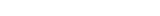for some natural number n. The number n is called the cardinality of the set, and is denoted |S|. (Note that the empty set
Empty set
In mathematics, and more specifically set theory, the empty set is the unique set having no elements; its size or cardinality is zero. Some axiomatic set theories assure that the empty set exists by including an axiom of empty set; in other theories, its existence can be deduced...

is considered finite, with cardinality zero.) If a set is finite, its elements may be written as a sequence
Sequence
In mathematics, a sequence is an ordered list of objects . Like a set, it contains members , and the number of terms is called the length of the sequence. Unlike a set, order matters, and exactly the same elements can appear multiple times at different positions in the sequence...

: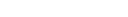In combinatorics
Combinatorics
Combinatorics is a branch of mathematics concerning the study of finite or countable discrete structures. Aspects of combinatorics include counting the structures of a given kind and size , deciding when certain criteria can be met, and constructing and analyzing objects meeting the criteria ,...

, a finite set with n elements is sometimes called an n-set
N-set
In mathematics, an n-set is a set containing exactly n elements, where n is a natural number. Thus, every finite set is an n-set for some specific natural number n. If S is any set, then a subset of S containing k elements is called a k-subset, or a k-combination...

. For example, the set {5,6,7} is a 3-set, a finite set with three elements.

## Basic properties

Any proper subset
Subset
In mathematics, especially in set theory, a set A is a subset of a set B if A is "contained" inside B. A and B may coincide. The relationship of one set being a subset of another is called inclusion or sometimes containment...

of a finite set S is finite and has fewer elements than S itself. As a consequence, there cannot exist a bijection
Bijection
A bijection is a function giving an exact pairing of the elements of two sets. A bijection from the set X to the set Y has an inverse function from Y to X. If X and Y are finite sets, then the existence of a bijection means they have the same number of elements...

between a finite set S and a proper subset of S. Any set with this property is called Dedekind-finite. Using the standard ZFC
Zermelo–Fraenkel set theory
In mathematics, Zermelo–Fraenkel set theory with the axiom of choice, named after mathematicians Ernst Zermelo and Abraham Fraenkel and commonly abbreviated ZFC, is one of several axiomatic systems that were proposed in the early twentieth century to formulate a theory of sets without the paradoxes...

axioms for set theory
Set theory
Set theory is the branch of mathematics that studies sets, which are collections of objects. Although any type of object can be collected into a set, set theory is applied most often to objects that are relevant to mathematics...

, every Dedekind-finite set is also finite, but this requires the axiom of choice (or at least the axiom of dependent choice
Axiom of dependent choice
In mathematics, the axiom of dependent choices, denoted DC, is a weak form of the axiom of choice which is still sufficient to develop most of real analysis...

).

Any injective function
Injective function
In mathematics, an injective function is a function that preserves distinctness: it never maps distinct elements of its domain to the same element of its codomain. In other words, every element of the function's codomain is mapped to by at most one element of its domain...

between two finite sets of the same cardinality is also a surjection
Surjective function
In mathematics, a function f from a set X to a set Y is surjective , or a surjection, if every element y in Y has a corresponding element x in X so that f = y...

, and similarly any surjection between two finite sets of the same cardinality is also an injection.

The union
Union (set theory)
In set theory, the union of a collection of sets is the set of all distinct elements in the collection. The union of a collection of sets S_1, S_2, S_3, \dots , S_n\,\! gives a set S_1 \cup S_2 \cup S_3 \cup \dots \cup S_n.- Definition :...

of two finite sets is finite, with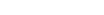In fact: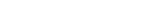More generally, the union of any finite number of finite sets is finite. The Cartesian product
Cartesian product
In mathematics, a Cartesian product is a construction to build a new set out of a number of given sets. Each member of the Cartesian product corresponds to the selection of one element each in every one of those sets...

of finite sets is also finite, with: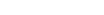Similarly, the Cartesian product of finitely many finite sets is finite. A finite set with n elements has 2n distinct subsets. That is, the
power set of a finite set is finite, with cardinality 2n.

Any subset of a finite set is finite. The set of values of a function when applied to elements of a finite set is finite.

All finite sets are countable, but not all countable sets are finite. (However, some authors use "countable" to mean "countably infinite", and thus do not consider finite sets to be countable.)

The free semilattice over a finite set is the set of its non-empty subsets, with the join operation being given by set union.

## Necessary and sufficient conditions for finiteness

In Zermelo–Fraenkel set theory
Zermelo–Fraenkel set theory
In mathematics, Zermelo–Fraenkel set theory with the axiom of choice, named after mathematicians Ernst Zermelo and Abraham Fraenkel and commonly abbreviated ZFC, is one of several axiomatic systems that were proposed in the early twentieth century to formulate a theory of sets without the paradoxes...

(ZF), the following conditions are all equivalent:
1. S is a finite set. That is, S can be placed into a one-to-one correspondence with the set of those natural numbers less than some specific natural number.
2. (Kazimierz Kuratowski
Kazimierz Kuratowski
Kazimierz Kuratowski was a Polish mathematician and logician. He was one of the leading representatives of the Warsaw School of Mathematics.-Biography and studies:...

) S has all properties which can be proved by mathematical induction beginning with the empty set and adding one new element at a time. (See the section on foundational issues for the set-theoretical formulation of Kuratowski finiteness.)
3. (Paul Stäckel
Paul Stäckel
Paul Gustav Samuel Stäckel was a German mathematician, active in the areas of differential geometry, number theory, and non-Euclidean geometry. In the area of prime number theory, he used the term twin prime for the first time.-External links:...

) S can be given a total ordering which is well-order
Well-order
In mathematics, a well-order relation on a set S is a strict total order on S with the property that every non-empty subset of S has a least element in this ordering. Equivalently, a well-ordering is a well-founded strict total order...

ed both forwards and backwards. That is, every non-empty subset of S has both a least and a greatest element in the subset.
4. Every one-to-one function from P(P(S)) into itself is onto. That is, the powerset of the powerset of S is Dedekind-finite (see below).
5. Every surjective function from P(P(S)) onto itself is one-to-one.
6. (Alfred Tarski
Alfred Tarski
Alfred Tarski was a Polish logician and mathematician. Educated at the University of Warsaw and a member of the Lwow-Warsaw School of Logic and the Warsaw School of Mathematics and philosophy, he emigrated to the USA in 1939, and taught and carried out research in mathematics at the University of...

) Every non-empty family of subsets of S has a minimal element with respect to inclusion.
7. S can be well-ordered and any two well-orderings on it are order isomorphic. In other words, the well-orderings on S have exactly one order type
Order type
In mathematics, especially in set theory, two ordered sets X,Y are said to have the same order type just when they are order isomorphic, that is, when there exists a bijection f: X → Y such that both f and its inverse are monotone...

.

If the axiom of choice is also assumed (the axiom of countable choice
Axiom of countable choice
The axiom of countable choice or axiom of denumerable choice, denoted ACω, is an axiom of set theory, similar to the axiom of choice. It states that any countable collection of non-empty sets must have a choice function...

is sufficient), then the following conditions are all equivalent:
1. S is a finite set.
2. (Richard Dedekind
Richard Dedekind
Julius Wilhelm Richard Dedekind was a German mathematician who did important work in abstract algebra , algebraic number theory and the foundations of the real numbers.-Life:...

) Every one-to-one function from S into itself is onto.
3. Every surjective function from S onto itself is one-to-one.
4. S is empty or every partial ordering of S contains a maximal element
Maximal element
In mathematics, especially in order theory, a maximal element of a subset S of some partially ordered set is an element of S that is not smaller than any other element in S. The term minimal element is defined dually...

.

## Foundational issues

Georg Cantor
Georg Cantor
Georg Ferdinand Ludwig Philipp Cantor was a German mathematician, best known as the inventor of set theory, which has become a fundamental theory in mathematics. Cantor established the importance of one-to-one correspondence between the members of two sets, defined infinite and well-ordered sets,...

initiated his theory of sets in order to provide a mathematical treatment of infinite sets. Thus the distinction between the finite and the infinite lies at the core of set theory. Certain foundationalists, the strict finitists
Finitism
In the philosophy of mathematics, one of the varieties of finitism is an extreme form of constructivism, according to which a mathematical object does not exist unless it can be constructed from natural numbers in a finite number of steps...

, reject the existence of infinite sets and thus advocate a mathematics based solely on finite sets. Mainstream mathematicians consider strict finitism too confining, but acknowledge its relative consistency: the universe of hereditarily finite set
Hereditarily finite set
In mathematics and set theory, hereditarily finite sets are defined recursively as finite sets consisting of 0 or more hereditarily finite sets.-Formal definition:...

s constitutes a model of Zermelo-Fraenkel set theory with the Axiom of Infinity
Axiom of infinity
In axiomatic set theory and the branches of logic, mathematics, and computer science that use it, the axiom of infinity is one of the axioms of Zermelo-Fraenkel set theory...

replaced by its negation.

Even for those mathematicians who embrace infinite sets, in certain important contexts, the formal distinction between the finite and the infinite can remain a delicate matter. The difficulty stems from Gödel's incompleteness theorems
Gödel's incompleteness theorems
Gödel's incompleteness theorems are two theorems of mathematical logic that establish inherent limitations of all but the most trivial axiomatic systems capable of doing arithmetic. The theorems, proven by Kurt Gödel in 1931, are important both in mathematical logic and in the philosophy of...

. One can interpret the theory of hereditarily finite sets within Peano arithmetic (and certainly also vice-versa), so the incompleteness of the theory of Peano arithmetic implies that of the theory of hereditarily finite sets. In particular, there exists a plethora of so-called non-standard models of both theories. A seeming paradox, non-standard models of the theory of hereditarily finite sets contain infinite sets --- but these infinite sets look finite from within the model. (This can happen when the model lacks the sets or functions necessary to witness the infinitude of these sets.) On account of the incompleteness theorems, no first-order predicate, nor even any recursive scheme of first-order predicates, can characterize the standard part of all such models. So, at least from the point of view of first-order logic, one can only hope to characterize finiteness approximately.

More generally, informal notions like set, and particularly finite set, may receive interpretations across a range of formal system
Formal system
In formal logic, a formal system consists of a formal language and a set of inference rules, used to derive an expression from one or more other premises that are antecedently supposed or derived . The axioms and rules may be called a deductive apparatus...

s varying in their axiomatics and logical apparatus. The best known axiomatic set theories include Zermelo-Fraenkel set theory (ZF), Zermelo-Fraenkel set theory with the Axiom of Choice (ZFC), Von Neumann–Bernays–Gödel set theory
Von Neumann–Bernays–Gödel set theory
In the foundations of mathematics, von Neumann–Bernays–Gödel set theory is an axiomatic set theory that is a conservative extension of the canonical axiomatic set theory ZFC. A statement in the language of ZFC is provable in NBG if and only if it is provable in ZFC. The ontology of NBG includes...

(NBG), Non-well-founded set theory
Non-well-founded set theory
Non-well-founded set theories are variants of axiomatic set theory which allow sets to contain themselves and otherwise violate the rule of well-foundedness...

, Bertrand Russell
Bertrand Russell
Bertrand Arthur William Russell, 3rd Earl Russell, OM, FRS was a British philosopher, logician, mathematician, historian, and social critic. At various points in his life he considered himself a liberal, a socialist, and a pacifist, but he also admitted that he had never been any of these things...

's Type theory
Type theory
In mathematics, logic and computer science, type theory is any of several formal systems that can serve as alternatives to naive set theory, or the study of such formalisms in general...

and all the theories of their various models. One may also choose among classical first-order logic, various higher-order logics and intuitionistic logic
Intuitionistic logic
Intuitionistic logic, or constructive logic, is a symbolic logic system differing from classical logic in its definition of the meaning of a statement being true. In classical logic, all well-formed statements are assumed to be either true or false, even if we do not have a proof of either...

.

A formalist
Formalism (mathematics)
In foundations of mathematics, philosophy of mathematics, and philosophy of logic, formalism is a theory that holds that statements of mathematics and logic can be thought of as statements about the consequences of certain string manipulation rules....

might see the meaning of set varying from system to system. A Platonist might view particular formal systems as approximating an underlying reality.

## Set-theoretic definitions of finiteness

In contexts where the notion of natural number
Natural number
In mathematics, the natural numbers are the ordinary whole numbers used for counting and ordering . These purposes are related to the linguistic notions of cardinal and ordinal numbers, respectively...

sits logically prior to any notion of set, one can define a set S as finite if S admits a bijection
Bijection
A bijection is a function giving an exact pairing of the elements of two sets. A bijection from the set X to the set Y has an inverse function from Y to X. If X and Y are finite sets, then the existence of a bijection means they have the same number of elements...

to some set of natural numbers of the form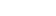. Mathematicians more typically choose to ground notions of number in set theory
Set theory
Set theory is the branch of mathematics that studies sets, which are collections of objects. Although any type of object can be collected into a set, set theory is applied most often to objects that are relevant to mathematics...

, for example they might model natural numbers by the order types of finite well-ordered sets. Such an approach requires a structural definition of finiteness that does not depend on natural numbers.

Interestingly, various properties that single out the finite sets among all sets in the theory ZFC turn out logically inequivalent in weaker systems such as ZF or intuitionistic set theories. Two definitions feature prominently in the literature, one due to Richard Dedekind
Richard Dedekind
Julius Wilhelm Richard Dedekind was a German mathematician who did important work in abstract algebra , algebraic number theory and the foundations of the real numbers.-Life:...

, the other to Kazimierz Kuratowski
Kazimierz Kuratowski
Kazimierz Kuratowski was a Polish mathematician and logician. He was one of the leading representatives of the Warsaw School of Mathematics.-Biography and studies:...

(Kuratowski's is the definition used above).

A set S is called Dedekind infinite if there exists an injective, non-surjective function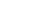. Such a function exhibits a bijection between S and a proper subset of S, namely the image of f. Given a Dedekind infinite set S, a function f, and an element x that is not in the image of f, we can form an infinite sequence of distinct elements of S, namely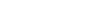. Conversely, given a sequence in S consisting of distinct elements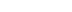, we can define a function f such that on elements in the sequence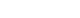and f behaves like the identity function otherwise. Thus Dedekind infinite sets contain subsets that correspond bijectively with the natural numbers. Dedekind finite naturally means that every injective self-map is also surjective.

Kuratowski finiteness is defined as follows. Given any set S, the binary operation of union endows the powerset P(S) with the structure of a semi-lattice. Writing K(S) for the sub-semi-lattice generated by the empty set
Empty set
In mathematics, and more specifically set theory, the empty set is the unique set having no elements; its size or cardinality is zero. Some axiomatic set theories assure that the empty set exists by including an axiom of empty set; in other theories, its existence can be deduced...

and the singletons, call set S Kuratowski finite if S itself belongs to K(S). Intuitively, K(S) consists of the finite subsets of S. Crucially, one does not need induction, recursion or a definition of natural numbers to define generated by since one may obtain K(S) simply by taking the intersection of all sub-semi-lattices containing the empty set and the singletons.

Readers unfamiliar with semi-lattices and other notions of abstract algebra may prefer an entirely elementary formulation. Kuratowski finite means S lies in the set K(S), constructed as follows. Write M for the set of all subsets X of P(S) such that:
• X contains the empty set;
• For every set T in P(S), if X contains T then X also contains the union of T with any singleton.

Then K(S) may be defined as the intersection of M.

In ZF, Kuratowski finite implies Dedekind finite, but not vice-versa. In the parlance of a popular pedagogical formulation, when the axiom of choice fails badly, one may have an infinite family of socks with no way to choose one sock from more than finitely many of the pairs. That would make the set of such socks Dedekind finite: there can be no infinite sequence of socks, because such a sequence would allow a choice of one sock for infinitely many pairs by choosing the first sock in the sequence. However, Kuratowski finiteness would fail for the same set of socks.